# Wave Walker DSP

## DSP Algorithms for RF Systems

DSP for Beginners: Simple Explanations for Complex Numbers! The second edition includes a new chapter on complex sinusoids.

Product to Sum Trigonometric Identities with Euler’s Formula
June 15, 2022

#### Introduction

This blog derives the product to sum trigonometric identities using Euler’s formula,

(1)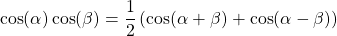(2)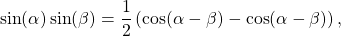(3)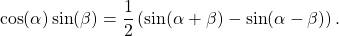More blogs on DSP Math:

#### cos(a) cos(b)

Start by substituting Euler’s formula in for both cosine terms,

(4)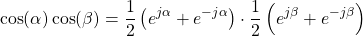Multiplying the right hand terms of (4),

(5)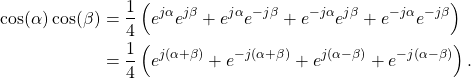Simplifying (5) with Euler’s formula results in

(6)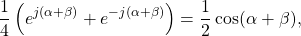(7)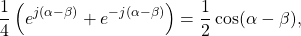such that

(8)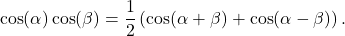#### sin(a) sin(b)

Start by substituting Euler’s formula in for both sine terms,

(9)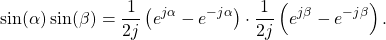Multiplying the right hand terms of (9),

(10)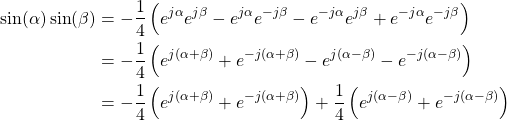Simplifying (10) with Euler’s formula results in

(11)(12)such that

(13)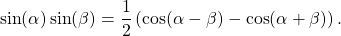#### cos(a) sin(b)

Start by substituting Euler’s formula in for cosine and sine,

(14)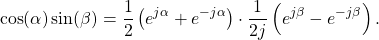Multiplying the right hand terms of (14),

(15)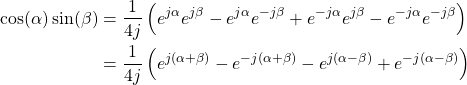Simplifying (15) with Euler’s formula results in

(16)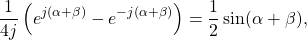(17)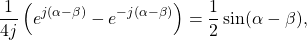such that

(18)#### Conclusion

The product to sum trigonometric identities were proven using Euler’s formula.

More blogs on DSP Math:

God, the Lord, is my strength; he makes my feet like the deer’s; he makes me tread on my high places. Habakkuk 3:19

This website participates in the Amazon Associates program. As an Amazon Associate I earn from qualifying purchases.